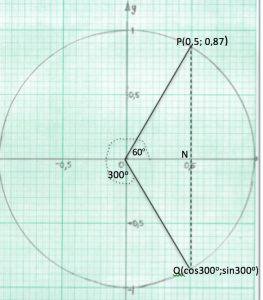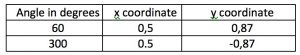DO NOT USE CALCULATORS FOR THIS WHOLE CLASS ACTIVITY.

YOU NEED TO KNOW: that the cosine and sine functions for ALL ANGLES are given by the coordinates of points on the UNIT CIRCLE. The x-coordinates of points on the unit circle give the cosine of the angle between the x-axis and the radius measured anti-clockwise and the y-coordinates give the sine of the angle.

In this activity learners will share the work of taking readings of the coordinates of points on the unit circle for the angles $$10^o, 20^o , …, 350^o, 360^o$$. Then you will plot these points to discover what the graphs of the cosine and sine functions look like.

STEP 1 On Sheet 1 use a protractor to measure an angle of 60o anti-clockwise from the x-axis and draw the lines OP and PNQ. The right angled triangle OPN has edges OP=1, ON=cos60o and PN=sin60o. From the lines on the grid you should see clear steps of 0,1 from -1 to + 1 in both x and y directions so you can take readings to 2 decimal places. Read the coordinates of P from the grid.

For 300o the radius is OQ and the readings are (cos300o; sin300o) =(0,5;-0,87) Note the sign. Readings should be recorded in a table like this.

STEP 2 Draw accurately the 6 angles on your card in this way, then take readings from the grid of the x-coordinates for cosines and the y-coordinates for sines correct to 2 decimal places, and to fill in the tables on your cards.

STEP 3 As a class fill in Sheet 2 using the results from the whole class. Several learners will have taken each reading so you can check that the readings are correct.

STEP 4 The next step is to plot the points (A, cos A) using Output 1 from Sheet 2.  ONLY THE FIRST TWO COLUMNS ARE USED FOR cos A. You could cover the Output 2 column for sinA or fold sheet 2 to hide that column.

On Sheet 3 plot on the horizontal axis the values of angle A in degrees from the 1st column and on the vertical axis the values of cos A from the 2nd column.

This graph of f(A)= cosA should be a NICE ROUNDED CURVE.

STEP 5 Plot the points (A, sinA) of the function g(A)= sin A on Sheet 3 using Output 2 from sheet 2. Plot on the horizontal axis the values of angle A in degrees from the 1st column and, on the vertical axis, the values of sin A from the 3rd Again the graph should be a NICE ROUNDED CURVE.

STEP 6 What do you notice when you compare the graphs of f(A) = cos A and g(A) = sin A?

What do you think happens if you go on to 370o, 380o, and so on and on ?

What happens for negative angles?